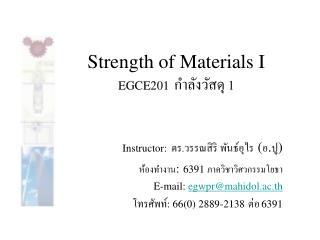DownloadDownload PresentationStrength of Materials I EGCE201 กำลังวัสดุ 1

# Strength of Materials I EGCE201 กำลังวัสดุ 1

Télécharger la présentation## Strength of Materials I EGCE201 กำลังวัสดุ 1

- - - - - - - - - - - - - - - - - - - - - - - - - - - E N D - - - - - - - - - - - - - - - - - - - - - - - - - - -
##### Presentation Transcript

1. Strength of Materials I EGCE201กำลังวัสดุ 1 Instructor: ดร.วรรณสิริ พันธ์อุไร (อ.ปู) ห้องทำงาน:6391 ภาควิชาวิศวกรรมโยธา E-mail: egwpr@mahidol.ac.th โทรศัพท์: 66(0) 2889-2138ต่อ6391

2. x x Recall the curvature equation The slope (q) & deflection (y) at any spanwise location can be derived Beam Deflections As a beam is loaded, • different regions are subjected to V and M • the beam will deflect

3. A cantilever with a point load • The moment is M(x) = -Px • The curvature equation for this case is • The curvature is at A and finite at B • Obviously, the curvature can be related to displacement

4. The slope & deflection equation • The following relations are established • For small angles,

5. Double Integration method • Multiply both sides of this equation by EI and integrate Slope eqn • Where C1 is a constant of integration, Integrating again Deflection eqn C1 and C2 must be determined from boundary conditions

6. Cantilever beam Overhanging beam Simply supported beam Boundary conditions

7. Statically indeterminate beam • The reactions at the supports cannot be completely determined from the 3 eqns. • The degreeto which a beam is statically indeterminate is identified by a no. of reactions that can’t be determined. • To determine all reactions, the beam deformations must be considered.

8. Statically indeterminate to 1st degree • From the FBD shown, one can write • Obviously not enough to solve for Ay, MA, and B

9. Begin by drawing a FBD diagram for an arbitrary segment of beam (1) For beam segment AC, summing moment about C we write The curvature equation is determined from Integrating twice in x, we have

10. Using the B.C.s x=0, q=0, and y=0 the constants C1 = C2 = 0 Using the 3rd B.C. (x=L, y=0) the above equation becomes or (2)

11. The 2 equations generated from the original FBD of the beam and the equation generated by considering a BC at x=L from the curvature equation: 2 equations and 2 unknowns, they are now solved to determine the reactions at all supports Ans

12. Statically indeterminate to 2nd degree • From the FBD shown, one can write • Obviously not enough to solve for Ay, MA, By and MB

13. For beam segment AC, summing moment about C we write The curvature equation is determined from Integrating twice in x, we have

14. Using the B.C.s x=0, q=0, and y=0 the constants C1 = C2 = 0 Using the 3rd B.C. (x=L, q=0, y=0) the above equation becomes or

15. 2 equations and 2 unknowns, they are now solved to determine the reactions at all supports Ans

16. Superposition • The slope & deflection at any point of the beam may be determined by superposing the slopes and deflections caused by the distributed and concentrated loads.

17. Superposition (cont.)

18. Superposition (cont.)

19. Superposition (cont.) • The method of superposition can be used for many loading conditions, provided the equations for the slope and deflection associated with a specific type of load are known. For example,

20. Tables • Click on each beam in which u are interested to see the curvature equation, max deflection, and slope for each loading condition (You should start working on it before seeing!)

21. Singularity functions • Instead of using the curvature equation, one can also use what so called the singularity functions. For the beam shown above, the bending moment in terms of singularity functions by

22. Integrate twice to get

23. Next Week 1. Quiz 2. Continuation of Beam Deflections - theorems of area moment method - moment diagrams by parts